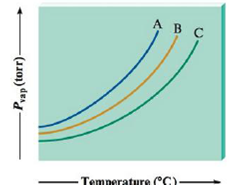Chapter 10, Problem 115AE

Chapter
Section
Textbook Problem

Consider the following vapor pressure versus temperature plot for three different substances: A, B, and C.If the three substances are CH4, SiH4, and NH3, match each curve to the correct substance.

Interpretation Introduction

Interpretation: In plot, given substance to be matched with corresponding curve.

Concept introduction

At given temperature, the vapor is elevated by pressure in thermodynamic equilibrium with its solid or liquid phase.

Explanation

Explanation

Record the given data

The plot is recorded as shown.

To explain the compounds which are matched with corresponding curves.

At any temperature, the plot shows that compound A has a high vapour pressure than compound B, with compound C having the lowest vapor pressure. Therefore, the sub compound with the weakest intermolecular forces is A, and the compound with the strongest intermolecular forces is C.

NH3 could form hydrogen-bonding interactions, whereas the others cannot

Still sussing out bartleby?

Check out a sample textbook solution.

See a sample solution

The Solution to Your Study Problems

Bartleby provides explanations to thousands of textbook problems written by our experts, many with advanced degrees!

Get Started